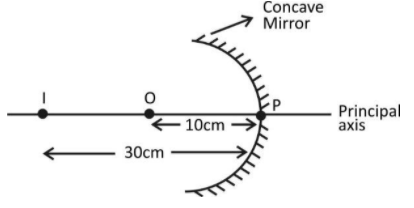Courses
Courses for Kids
Free study material
Free LIVE classes
MoreLIVE
Join Vedantu’s FREE Mastercalss

# A concave mirror produces three times magnified real images of an object placed at $10cm$ in front of it. Where is the image located?Verified
330k+ views
Hint: We know concave mirror is also known as converging mirror. If the image formed is real then it is always inverted. Also we have to keep in mind the sign conventions while doing the calculations that are all the distance to the left of the pole are taken negative.

Complete step by step solution:The mirror formula is given as $\dfrac{1}{v}+\dfrac{1}{u}=\dfrac{1}{f}$ and magnification is gives as, $m=\dfrac{height\,\,of\,\,image}{Height\,\,of\,\,object}$
In concave mirror the real image is always inverted so by the question, magnification $m=-3,\,\,also\,\,u=-10cm,\,v=?$
We know that formula for magnification is spherical mirror’s is $m=\dfrac{-v}{u}$
Putting the values of $m=-3$ and $u=-10\,\,in\,\,m=\dfrac{-v}{u}$,

Therefore, $-3=-\dfrac{v}{(-10)}$
$v=-30cm$
I$\to$ Image
O$\to$ Object
P$\to$ Pole

Note: Basic sign convention for the spherical mirror are
1. If a virtual image is formed (i.e. behind the mirror) then $v$ is positive.
2. If a real image is formed (i.e. in front of mirror) then $v$ is negative.
3. Object distance $u$ is always negative in the case of a spherical mirror as it is on the left side of the mirror.
4. Focal length of the concave mirror is negative. It is because focus is in front of mirror
5. Focal length of the convex mirror is positive. It is because focus is behind the mirror.
6. In magnification formula of $m=\dfrac{-v}{u}$ for spherical mirrors, if $m$ is positive, it means image formed is virtual and erect and if $m$ is negative it means image formed is real and inverted.
Last updated date: 02nd Oct 2023
Total views: 330k
Views today: 5.30k